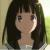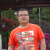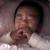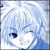## 用php实现约瑟夫环问题，求指导

为什么80%的码农都做不了架构师？->>>```<?php
function getking(\$n,\$m){
\$array=range(1,\$n);
\$now=0;//初始化\$now，为定位标志，也就是从它开始数
\$nn=\$n;//不破坏初始量
while(\$nn>1){
\$now=(\$now+\$m)%\$nn;//计算该第几个猴子出局了，因为是圈，取余就够了
if(!\$now){\$now=\$nn;}//如果正好整除那么这个猴子是最后一个（不是第0个）
array_splice(\$array,\$now-1,1);//去掉这个猴子
\$nn--;\$now--;//总数减少一个，标志前移一个
}
echo \$array;
}
getking(100,17);```

ps. array_splice是我无意间发现的函数，因为unset一个数组元素后不会重建键值，这个函数可以重建的。如果不是这样我就重新循环定义一个新数组了。

0```function kickMonkey (\$n,\$m) {
\$s = 0;
for (\$i=2; \$i<=\$n; \$i++) {
\$s = (\$s+\$m)%\$i;
}
\$win = \$s+1;
return \$win;
}```00